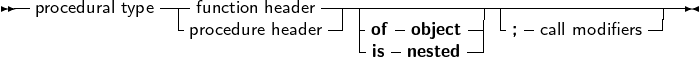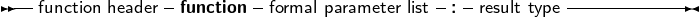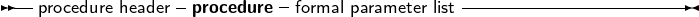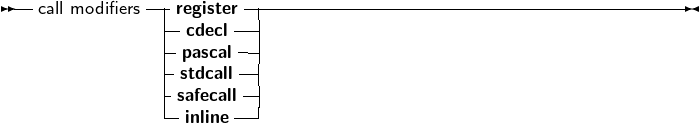### 3.6 Procedural types

Free Pascal has support for procedural types, although it differs a little from the Turbo Pascal or Delphi implementation of them. The type declaration remains the same, as can be seen in the following syntax diagram:

_________________________________________________________________________________________________________
Procedural types___________________________________________________________________

For a description of formal parameter lists, see chapter 14, page 750. The two following examples are valid type declarations:

```Type TOneArg = Procedure (Var X : integer);
TNoArg = Function : Real;
var proc : TOneArg;
func : TNoArg;
```

One can assign the following values to a procedural type variable:

1. Nil, for both normal procedure pointers and method pointers.

2. A variable reference of a procedural type, i. e. another variable of the same type.

3. A global procedure or function address, with matching function or procedure header and calling convention.

Given these declarations, the following assignments are valid:

```Procedure printit (Var X : Integer);
begin
WriteLn (x);
end;
...
Proc := @printit;
Func := @Pi;
```

From this example, the difference with Turbo Pascal is clear: In Turbo Pascal it isn’t necessary to use the address operator (@) when assigning a procedural type variable, whereas in Free Pascal it is required. In case the -MDelphi or -MTP switches are used, the address operator can be dropped.

Remark The modifiers concerning the calling conventions must be the same as the declaration; i. e. the following code would give an error:

```Type TOneArgCcall = Procedure (Var X : integer);cdecl;
var proc : TOneArgCcall;
Procedure printit (Var X : Integer);
begin
WriteLn (x);
end;
begin
Proc := @printit;
end.
```

Because the TOneArgCcall type is a procedure that uses the cdecl calling convention.

In case the is nested modified is added, then the procedural variable can be used with nested procedures. This requires that the sources be compiled in macpas or ISO mode, or that the nestedprocvars modeswitch be activated:

```{\$modeswitch nestedprocvars}
program tmaclocalprocparam3;

type
tnestedprocvar = procedure is nested;

var
tempp: tnestedprocvar;

procedure p1( pp: tnestedprocvar);
begin
tempp:=pp;
tempp
end;

procedure p2( pp: tnestedprocvar);
var
localpp: tnestedprocvar;
begin
localpp:=pp;
p1( localpp)
end;

procedure n;
begin
writeln( 'calling through n')
end;

procedure q;

var qi: longint;

procedure r;
begin
if qi = 1 then
writeln( 'success for r')
else
begin
writeln( 'fail');
halt( 1)
end
end;

begin
qi:= 1;
p1( @r);
p2( @r);
p1( @n);
p2( @n);

end;

begin
q;
end.
```

In case one wishes to assign methods of a class to a variable of procedural type, the procedural type must be declared with the of object modifier.

The two following examples are valid type declarations for method procedural variables (also known as event handlers because of their use in GUI design):

```Type TOneArg = Procedure (Var X : integer) of object;
TNoArg = Function : Real of object;
var
oproc : TOneArg;
ofunc : TNoArg;
```

A method of the correct signature can be assigned to these functions. When called, Self will be pointing to the instance of the object that was used to assign the method procedure.

The following object methods can be assigned to oproc and ofunc:

```Type
TMyObject = Class(TObject)
Procedure DoX (Var X : integer);
Function DoY: Real;
end;

Var
M : TMyObject;

begin
oproc:=@M.DoX;
ofunc:=@M.DOY;
end;
```

When calling oproc and ofunc, Self will equal M.

This mechanism is sometimes called Delegation.

Remark When comparing two variables of type method, only the method’s address is compared, not the instance pointer. That means that the following program will print True:

```Type
TSomeMethod = Procedure  of object;

TMyObject = Class(TObject)
Procedure DoSomething;
end;

Procedure TMyObject.DoSomething;

begin
Writeln('In DoSomething');
end;

var
X,Y : TMyObject;
P1,P2 : TSomeMethod;

begin
X:=TMyObject.Create;
Y:=TMyObject.Create;
P1:=@X.DoSomething;
P2:=@Y.DoSomething;
Writeln('Same method : ',P1=P2);
end.
```

If both pointers must be compared, a typecast to TMethod must be done, and both pointers should be compared. TMethod is defined in the system unit as follows:

```TMethod = record
Code : CodePointer;
Data : Pointer;
end;
```

The following program will therefore print False:

```Type
TSomeMethod = Procedure  of object;

TMyObject = Class(TObject)
Procedure DoSomething;
end;

Procedure TMyObject.DoSomething;

begin
Writeln('In DoSomething');
end;

var
X,Y : TMyObject;
P1,P2 : TMethod;

begin
X:=TMyObject.Create;
Y:=TMyObject.Create;
P1:=TMethod(@X.DoSomething);
P2:=TMethod(@Y.DoSomething);
Writeln('Same method : ',(P1.Data=P2.Data) and (P1.Code=P1.Code));
end.
```